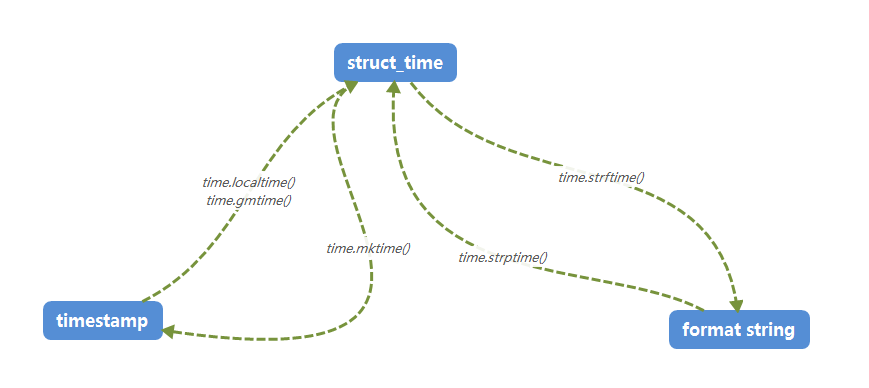# 一、日期时间的表示方法：

• 时间戳 timestamp：

• 简介：时间戳表示的是从1970年1月1日00:00:00开始按秒计算的偏移量，是一个float类型
• 展示形式：1575278720.331

• 时间元组 struct_time：

• 简介：共9个元素，时间戳和格式化时间转换的桥梁
• 展现形式：time.struct_time(tm_year=2019, tm_mon=12, tm_mday=2, tm_hour=17, tm_min=25, tm_sec=0, tm_wday=0, tm_yday=336, tm_isdst=0)

• 格式化时间 format string

• 简介：格式化时间，已格式化的结构使时间更具可读性。包括自定义格式和固定格式。
• 展现形式：1111-11-11 1:11:11

# 二、时间格式转换图# 三、time模块

1、time.time()

>>> import time
>>> time.time()
1575285984.093

2、time.localtime()

localtime()  :本地时间

>>> time.localtime() # 不传参数
time.struct_time(tm_year=2019, tm_mon=12, tm_mday=2, tm_hour=19, tm_min=27, tm_sec=58, tm_wday=0, tm_yday=336, tm_isdst=0)

>>> time.localtime(time.time()) # 传入当前时间  1575286025.549
time.struct_time(tm_year=2019, tm_mon=12, tm_mday=2, tm_hour=19, tm_min=28, tm_sec=53, tm_wday=0, tm_yday=336, tm_isdst=0)

>>> time.localtime(1575286025.549)  # 传入其他时间
time.struct_time(tm_year=2019, tm_mon=12, tm_mday=2, tm_hour=19, tm_min=27, tm_sec=5, tm_wday=0, tm_yday=336, tm_isdst=0)

3、time.gmtime()

gmtime() : 世界标准时间

>>> time.gmtime()
time.struct_time(tm_year=2019, tm_mon=12, tm_mday=2, tm_hour=11, tm_min=33, tm_sec=29, tm_wday=0, tm_yday=336, tm_isdst=0)

4、time.gmtime()

>>> time.mktime(time.localtime())
1575286671.0

5、time.strftime(format[, t])

python中时间日期格式化符号：

• %y 两位数的年份表示（00-99）
• %Y 四位数的年份表示（000-9999）
• %m 月份（01-12）
• %d 月内中的一天（0-31）
• %H 24小时制小时数（0-23）
• %I 12小时制小时数（01-12）
• %M 分钟数（00=59）
• %S 秒（00-59）
• %a 本地简化星期名称
• %A 本地完整星期名称
• %b 本地简化的月份名称
• %B 本地完整的月份名称
• %c 本地相应的日期表示和时间表示
• %j 年内的一天（001-366）
• %p 本地A.M.或P.M.的等价符
• %U 一年中的星期数（00-53）星期天为星期的开始
• %w 星期（0-6），星期天为星期的开始
• %W 一年中的星期数（00-53）星期一为星期的开始
• %x 本地相应的日期表示
• %X 本地相应的时间表示
• %Z 当前时区的名称
• %% %号本身

>>> time.strftime(“%Y-%m-%d %X”,time.localtime())
‘2019-12-02 19:45:44’

6、time.strptime(string, format)

>>> time.strptime(“2019-12-02 19:45:44″,”%Y-%m-%d %X”)
time.struct_time(tm_year=2019, tm_mon=12, tm_mday=2, tm_hour=19, tm_min=45, tm_sec=44, tm_wday=0, tm_yday=336, tm_isdst=-1)

https://www.cnblogs.com/beard/p/11972046.html

「点点赞赏，手留余香」

还没有人赞赏，快来当第一个赞赏的人吧！
Python
TensorFlow
0 条回复 A 作者 M 管理员
所有的伟大，都源于一个勇敢的开始！